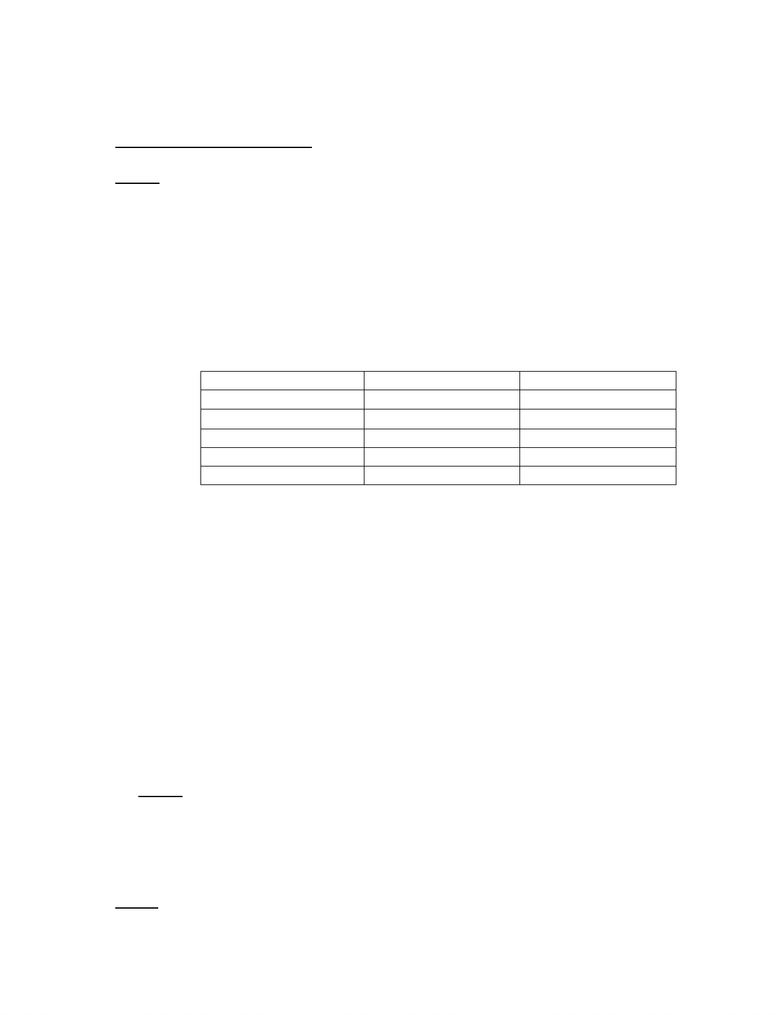Study Guides (390,000)
CA (150,000)
U of A (4,000)
CHEM (200)
CHEM101 (70)
Final

# CHEM101 Study Guide - Final Guide: Hygroscopy, Efflorescence, Flame Test

Department
Chemistry
Course Code
CHEM101
Professor
Yoram Apelblat
Study Guide
Final

This preview shows page 1. to view the full 4 pages of the document.Chemistry 101 Lab Final Notes
Definitions & Things to Know:
LAB A:
Spectrums/Continuous Spectrum (Silberberg, Fig. 7.3)
Discontinuous Spectrum… Discharge tubes (Fig. 7.8/7.9)
Emission of photons
Wavelength and energy of photons
o Spectrums are unique for each element
Light emission (p. 273)
o Flame test
o Fireworks
o Fluorescent lights (usually Hg discharge tubes)
Section 7.1 textbook
Constant
Symbol
Unit or Value
Wavelength
Meters
Frequency
Cycles per second, Hz
Speed of light
c
3.0 x 108 m/s
Planck’s Constant
h
6.626 x 10-34 J.s
Nuclear Charge
Z
 = c
Ephoton = h = hc/
SI units and prefixes
Bohr Model… (Silberberg p. 271)
o En = -Z2 RH / n2 where RH = 2.178 x 10-18 J
o Z is the nuclear charge (eg. H = 1, He = 2, Li = 3)
o A transition of the e- between level m to level n would result in
(p. 271)
E = (energy of final level) (energy of initial level) = En - Em
= - Z2 RH {(1/n2) (1/m2)}
Know how to operate a spectrometer (Fig B7.3 Silberberg)
The plot of A against wavelength is called a spectrum
LAB B:
Nomenclature (Section 2.8)
Stoichiometry (Chapter 3)
Balancing Chemical Reactions (Section 3.3)
Amphoteric (reacts with either an acid or base)… (p. 798)
LAB C: